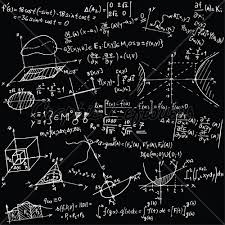# Expression 8151

For what values ​​of x is the value of the expression 5x + 11 equal to that of the expression 7x + 31?

x =  -10

### Step-by-step explanation:

5x + 11 = 7x + 31

5·x + 11 = 7·x + 31

2x = -20

x = -20/2 = -10

x = -10

Our simple equation calculator calculates it.Did you find an error or inaccuracy? Feel free to write us. Thank you!

Tips for related online calculators
Do you have a linear equation or system of equations and looking for its solution? Or do you have a quadratic equation?